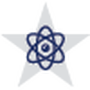1.### Lesson Plan: Concepts of Engineering Math

• Resource ID: 14893
In this lesson, students will apply engineering mathematics to various bridge applications, using team building strategies. This includes understanding different types of bridges, how to scale objects and using dimensional analysis.
2.### Lesson Plan: STEM - Inductive Reactance

• Resource ID: 14822
• Grade Range: 10, 11, 12
In this lesson, students will have an understanding of how inductive reactance impacts the operation of electrical equipment.
3.### Lesson Plan: AC/DC Electronics - Electrical Power

• Resource ID: 14827
• Grade Range: 10, 11, 12
In this lesson, students will be introduced to Ohm's law, Kirchoff's laws, and power laws to actual or simulated circuits. Students will apply these laws to construct and repair circuits.
4.### Lesson Plan: Engineering and Design Capstone Research Project 2

• Resource ID: 14871
In this lesson, students will learn to create a prototype that meets the criteria given in the Engineering Design Process/Problem Solving Grading Rubric. Students will learn to communicate ideas through the BLOG”ineering Design Process Website..
5.### Lesson Plan: Engineering Design Capstone Research Project Part 4

• Resource ID: 14875
• Grade Range: 10, 11, 12
In this lesson, students will learn and apply the knowledge in designing a product prototype, prepare and make a team presentation of their research and results that meets the criteria given in the Engineering Design PowerPoint or Prezi and Oral Presentation Rubric.
6.### Lesson Plan: Engineering Design Mini Project

• Resource ID: 14883
In this lesson, students will explain the concept of engineering design, apply engineering design concepts to a problem scenario, practice sketching technical drawings in their engineering notebooks, and reinforce collaborative and communication skills.
7.### Lesson Plan: Engineering Design Process

• Resource ID: 14918
• Grade Range: 10, 11, 12
In this lesson, students will demonstrate the steps of the design process in robotic applications.
8.### Lesson Plan: The Nature of Matter

• Resource ID: 14804
• Grade Range: 10, 11, 12
In this lesson, students will learn how matter is fundamental to the understanding of electricity. Student will also be able to describe the atomic models, electron orbits and shells, and how valence electrons determine conductivity.
9.### Lesson Plan: AC/DC - Electronic Hand Tools

• Resource ID: 14800
• Grade Range: 10, 11, 12
In this lesson, students will learn how to identify and maintain basic electric hand tools through hands-on experiences cleaning, lubricating, and adjusting a variety of tools.
10.### Lesson Plan: Magnetism

• Resource ID: 14802
• Grade Range: 10, 11, 12
In this lesson, students will learn about magnetism, which is fundamental to understanding electricity. This lesson includes a lab.
11.### Lesson Plan: AC/DC - Electrical Energy

• Resource ID: 14812
• Grade Range: 10, 11, 12
In this lesson, students will apply electrical theory to generators, electric motors, and transformer. Students will demonstrate the ability to use and test batteries.
12.### Lesson Plan: Resistance, Resistors - Understanding Color Codes

• Resource ID: 14831
• Grade Range: 10, 11, 12
In this lesson, students will learn to interpret the resistor color codes to determine the resistance and tolerance of a given resistor.
13.### Lesson Plan: Engineering Mathematics - Electrical Systems

• Resource ID: 14899
In this lesson, students will learn about electricity and design a building/wing/floor that meets a certain power consumption criteria using conventional power sources (fossil fuels) and renewable power sources.
14.### Engineering Design and Presentation II

• Grade Range: 9, 10, 11, 12, Postsecondary
Engineering Design and Presentation II is a continuation of knowledge and skills learned in Engineering Design and Presentation I. Students enrolled in this course will demonstrate knowledge and skills of the design process as it applies to engineering fields using multiple software applications...
15.### Engineering Science

• Grade Range: 9, 10, 11, 12, PostsecondaryEngineering Science is an engineering course designed to expose students to some of the major concepts and technologies that they will encounter...
16.### Principles of Technology

• Grade Range: 10, 11, 12In Principles of Technology, students will conduct laboratory and field investigations, use scientific methods during investigations, and make...
17.### Digital Electronics

• Grade Range: 9, 10, 11, 12, PostsecondaryDigital Electronics is the study of electronic circuits that are used to process and control digital signals. In contrast to analog electronics...
18.### Engineering Design and Problem Solving

• Grade Range: 9, 10, 11, 12, PostsecondaryThe Engineering Design and Problem-Solving course is the creative process of solving problems by identifying needs and then devising solutions. The...
19.### Robotics II

• Grade Range: 9, 10, 11, 12, PostsecondaryIn Robotics II, students will explore artificial intelligence and programming in the robotic and automation industry. Through implementation of...
20.### Engineering Mathematics

• Grade Range: 9, 10, 11, 12, PostsecondaryEngineering Mathematics is a course where students solve and model design problems. Students will use a variety of mathematical methods and...